# 卷积神经网络中的各种池化操作¶

1. 抑制噪声，降低信息冗余
2. 提升模型的尺度不变性、旋转不变形
3. 降低模型计算量
4. 防止过拟合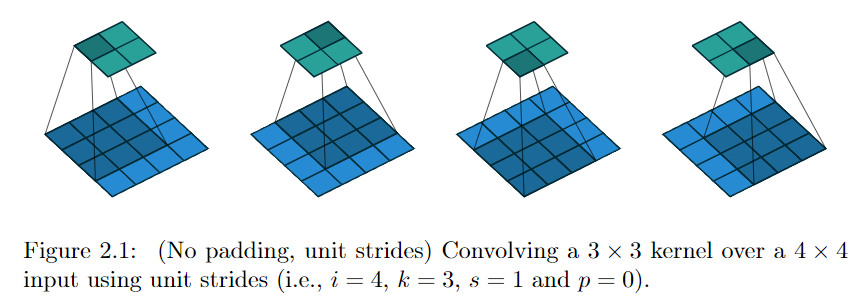o=\lfloor\frac{i-k}{s} \rfloor+1

### 1. 最大/平均池化¶

>>> import torch
>>> import torch.nn.functional as F
>>> input = torch.Tensor(4,3,16,16)
>>> output = F.max_pool2d(input, kernel_size=2, stride=2)
>>> output.shape
torch.Size([4, 3, 8, 8])
>>>


>>> import torch
>>> import torch.nn.functional as F
>>> input = torch.Tensor(4,3,16,16)
>>> output = F.avg_pool2d(input, kernel_size=2, stride=2)
>>> output.shape
torch.Size([4, 3, 8, 8])
>>>


### 2. 随机池化¶

Stochastic pooling如下图所示，特征区域的大小越大，代表其被选择的概率越高，比如左下角的本应该是选择7，但是由于引入概率，5也有一定几率被选中。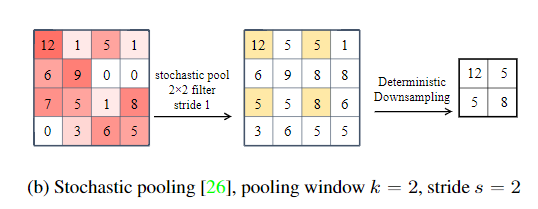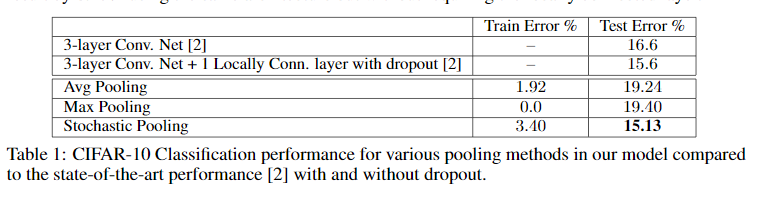### 4. 组合池化¶

def add_avgmax_pool2d(x, output_size=1):
return 0.5 * (x_avg + x_max)

def cat_avgmax_pool2d(x, output_size=1):


### 5. Spatial Pyramid Pooling¶

SPP是在SPPNet中提出的，SPPNet提出比较早，在RCNN之后提出的，用于解决重复卷积计算和固定输出的两个问题，具体方法如下图所示：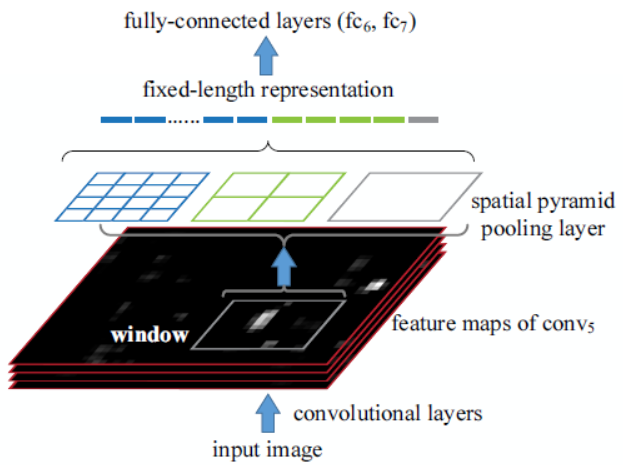### SPP ###
[maxpool]
stride=1
size=5

[route]
layers=-2

[maxpool]
stride=1
size=9

[route]
layers=-4

[maxpool]
stride=1
size=13

[route]
layers=-1,-3,-5,-6

### End SPP ###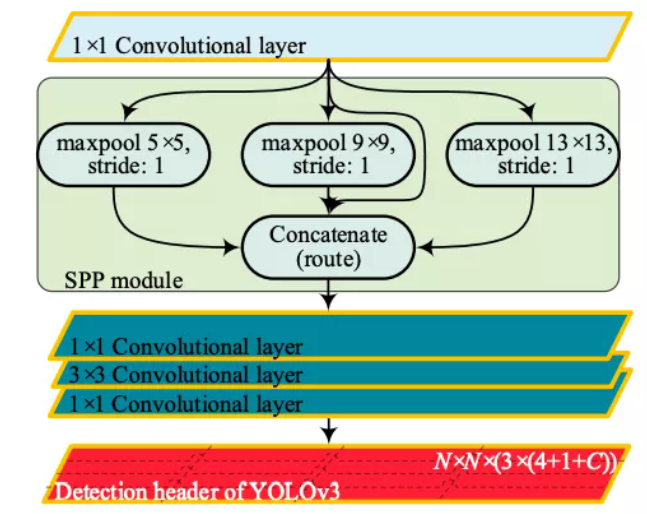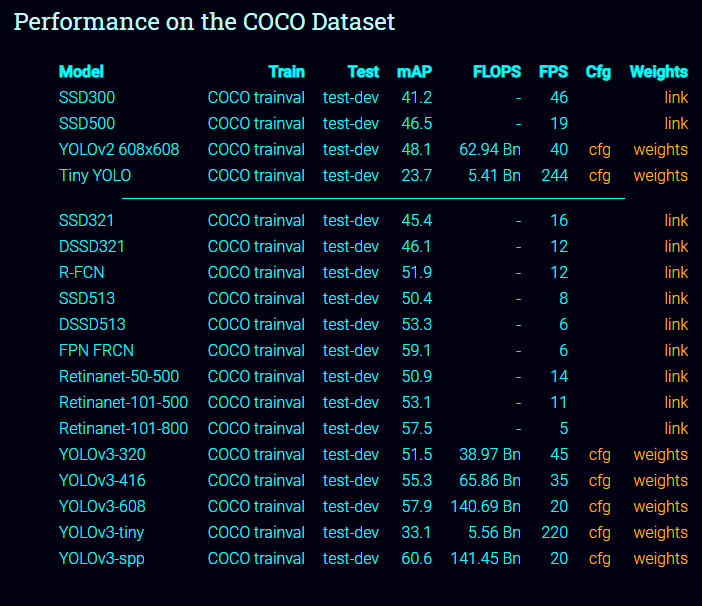1. 从感受野角度来讲，之前计算感受野的时候可以明显发现，maxpool的操作对感受野的影响非常大，其中主要取决于kernel size大小。在SPP中，使用了kernel size非常大的maxpool会极大提高模型的感受野，笔者没有详细计算过darknet53这个backbone的感受野，在COCO上有效很可能是因为backbone的感受野还不够大。
2. 第二个角度是从Attention的角度考虑，这一点启发自CSDN@小楞（链接在参考文献中），他在文章中这样讲：

Attention机制很多都是为了解决远距离依赖问题，通过使用kernel size接近特征图的size可以以比较小的计算代价解决这个问题。另外就是如果使用了SPP模块，就没有必要在SPP后继续使用其他空间注意力模块比如SK block，因为他们作用相似，可能会有一定冗余。

ps: 这个想法还没有进行试验的验证，有兴趣的可以将YOLOv3-spp中的kernel size改为19，然后在COCO数据集上测试，看是否能够超越60.6。

### 6. Global Average/Max Pooling¶

Gloabel Average Pooling 是NIN里边的做法，一般使用torchvision提供的预训练模型进行finetune的时候，通常使用Global Average Pooling，原因就是可以不考虑图片的输入尺寸，只与filter有关。

>>> import torch
>>> input = torch.zeros((4,12,18,18)) # batch size, fileter, h, w
>>> output = gap(input)
>>> output.shape
torch.Size([4, 12, 1, 1])
>>> output.view(input.shape,-1).shape
torch.Size([4, 12])
>>>


NetVLAD的一个输入是一个W x H x D的图像特征，例如VGG-Net最后的3 x 3 x 512这样的矩阵，在网络中还需加一个维度为Batchsize。

NetVLAD还需要另输入一个标量K即表示VLAD的聚类中心数量，它主要是来构成一个矩阵C，是通过原数据算出来的每一个$W \times H$特征的聚类中心，C的shape即$C: K \times D$，然后根据三个输入，VLAD是计算下式的V:

V(j, k) = \sum_{i=1}^{N}{a_k(x_i)(x_i(j) - c_k(j))}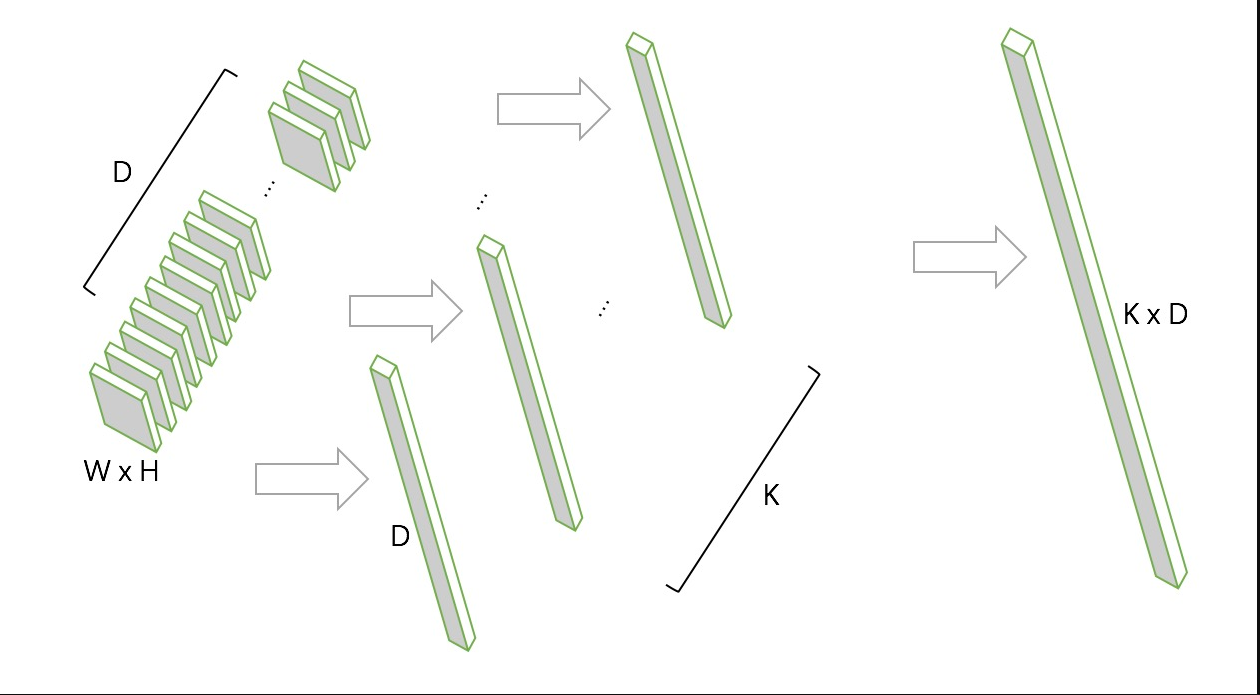a_k = \frac{e^{W_k^T x_i + b_k}}{e^{W_{k'}^T x_i + b_{k'}}}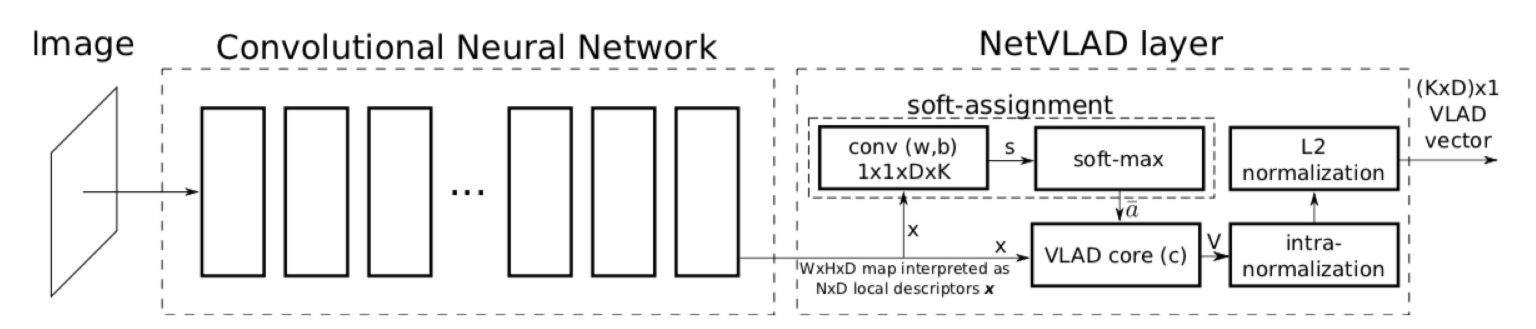### 8. 双线性池化¶

Bilinear Pooling是在《Bilinear CNN Models for Fine-grained Visual Recognition》被提出的，主要用在细粒度分类网络中。双线性池化主要用于特征融合，对于同一个样本提取得到的特征x和特征y, 通过双线性池化来融合两个特征(外积)，进而提高模型分类的能力。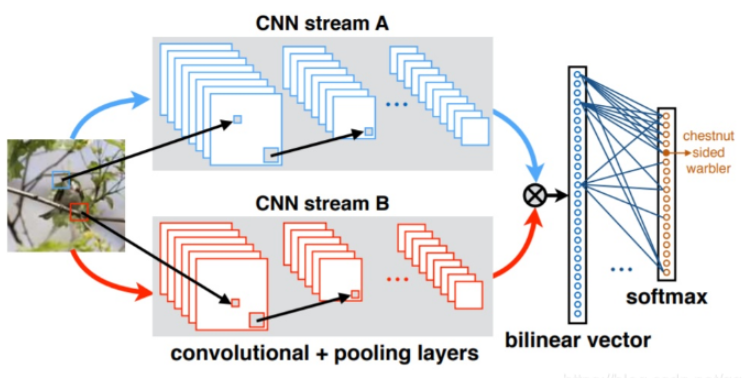pytorch实现：

X = torch.reshape(N, D, H * W)                        # Assume X has shape N*D*H*W
X = torch.bmm(X, torch.transpose(X, 1, 2)) / (H * W)  # Bilinear pooling
assert X.size() == (N, D, D)
X = torch.reshape(X, (N, D * D))
X = torch.sign(X) * torch.sqrt(torch.abs(X) + 1e-5)   # Signed-sqrt normalization
X = torch.nn.functional.normalize(X)                  # L2 normalization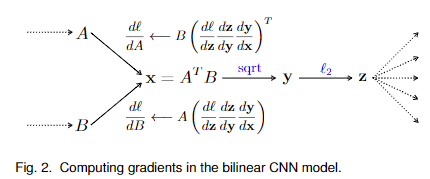https://zhuanlan.zhihu.com/p/62532887

## 9. UnPooling¶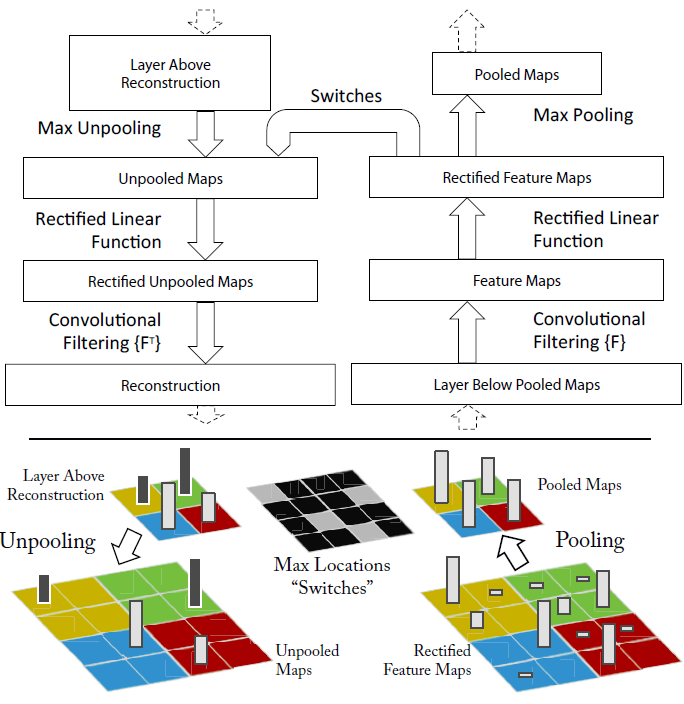1.在Pooling（一般是Max Pooling）时，保存最大值的位置。

2.中间经历若干网络层的运算。

3.上采样阶段，利用第1步保存的Max Location，重建下一层的feature map。

UnPooling不完全是Pooling的逆运算，Pooling之后的feature map，要经过若干运算，才会进行UnPooling操作；对于非Max Location的地方以零填充。然而这样并不能完全还原信息。

### 10. 参考¶

https://arxiv.org/pdf/1603.07285.pdf

https://arxiv.org/abs/1301.3557

https://zhuanlan.zhihu.com/p/77040467

https://arxiv.org/pdf/1611.05138.pdf

https://blog.csdn.net/qq_33270279/article/details/103898245

https://github.com/scutan90/DeepLearning-500-questions

https://zhuanlan.zhihu.com/p/62532887

https://blog.csdn.net/qq_32768091/article/details/84145088